Join one of our upcoming courses taking place around the world! Explore now

# Gravity Field Stress

The Gravity field stress option is used to define an in-situ stress field which varies with depth. Gravity field stress is used for surface or near-surface excavations.

The depth can be measured from either:

• A user-specified Ground Surface Elevation (useful if the ground profile is a single horizontal elevation).
• The actual ground surface of your model with the ground surface layer.

The depth and the unit weight of the overlying material determine the vertical stress distribution throughout the model. The horizontal stresses are then calculated by multiplying the vertical stress by the Horizontal/Vertical Stress Ratio(s).

In the Field Stress dialog, the following parameters are used to define a Gravity stress field.

## Ko Condition

When this checkbox is selected, this implies hydrostatic conditions where the horizontal stress is equal in all directions. In this case, only a single horizontal/vertical stress ratio needs to be defined (i.e. stress ratio K1). This is the default setting.

If the horizontal stress is dependent on direction, then clear the Ko Condition checkbox and you will be able to enter two orthogonal stress ratios (K1 and K2) and a direction (for K1). See below for more information.

## Ground Surface Elevation

The Ground Surface Elevation is the vertical coordinate of the ground surface with respect to your model axes. It is not the depth below the ground surface of your excavations. The Ground Surface Elevation is measured on the Z-axis.

• If you have selected the Use Actual Ground Surface checkbox, the Ground Surface Elevation option is not applicable and will be disabled. See below for more information.

## Unit Weight

The Unit Weight is a value of the unit weight of the gravity load applied with Ground Surface Elevation. It calculates the vertical component of the gravitational field stress at any point in the model.

## Use Actual Ground Surface

If the Use Actual Ground Surface checkbox is selected:

• The initial vertical stress at a given point is calculated using the depth below the actual ground surface of the model, and the actual unit weight of the overlying material(s) as entered in the Define Material Properties dialog.
• The Ground Surface Elevation and Unit Weight of Overburden options will be disabled.

The Use Actual Ground Surface option can be useful for surface excavations such as open-pit mines, slopes, rock cuts etc. The advantage of using this option is that it provides a better initial estimate of the vertical in-situ stress, for surface excavations where the ground surface is irregular and is not defined by a single horizontal surface.

The Use Actual Ground Surface option is also recommended if you are carrying out a slope stability analysis using the Shear Strength Reduction method. In general, it provides more reliable and accurate results with less computation, due to the more realistic estimate of the initial stress distribution.

## Use Variable Stress Ratio

By default, the horizontal/vertical stress ratio K is a constant value (i.e. horizontal stress is directly proportional to the vertical stress).

The Use Variable Stress Ratio checkbox allows you to define a variable stress ratio that is a function of depth z according to the following equation:

K = a + bzc

where:

K = horizontal / vertical stress ratio

z = vertical depth from ground surface

a, b, c = user-defined parameters

If you select this checkbox you can enter values of a, b and c to define the stress ratio(s) as a function of depth z according to this equation.

## Stress Ratios

The Horizontal/Vertical stress ratios (K1 and K2) are used to calculate the horizontal components of the gravitational field stress, based on the vertical stress at any point in the model.

• If the Ko Condition checkbox is selected (see above) then only a single stress ratio is required (K1).
• If the Ko Condition checkbox is not selected then you must define two orthogonal stress ratios (K1 and K2) and a direction for K1.
• The stress ratio(s) which you enter will apply to either the Total stress or Effective stress, according to the selection of the Use Effective Stress Ratio checkbox (see above).
• If the Use Variable Stress Ratio checkbox is selected then the stress ratio(s) can be defined as a function of depth (see above).

If the Use Effective Stress Ratio checkbox is not selected, then the horizontal stress is calculated from the total vertical stress, as follows: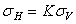where: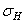= total horizontal stress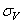= total vertical stress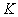= horizontal/vertical stress ratio K1 or K2 (total stress)

If the Use Effective Stress Ratio checkbox is selected, then the horizontal stress is calculated from the effective vertical stress, as follows: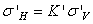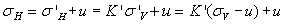where:= total horizontal stress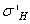= effective horizontal stress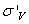= effective vertical stress= total vertical stress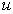= pore pressure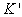= horizontal/vertical stress ratio K1 or K2 (effective stress)

### STRESS RATIO FOR SOILS

See the Theory section for a document (In-situ Stress States in Soils) that discusses the horizontal/vertical effective stress ratio for soils (the coefficient of earth pressure at rest), and various formulae that can be used to estimate a value of K for normally consolidated or overconsolidated soils.

### VARIABLE STRESS RATIO

If the Use Variable Stress Ratio checkbox is selected then the stress ratios can be defined as a function of depth, see above for details.

## Orientation of Horizontal Stress 1

If the Ko Condition checkbox is not selected then you must enter two orthogonal stress ratios K1 and K2 and a direction for K1. There are two options available for defining the Orientation of Horizontal Stress 1:

• Trend/Plunge - only a value of Trend is required (-360 to +360 degrees) since Plunge = 0
• Vector - you must enter XY vector components defining a vector in the horizontal plane.

## Locked In Stress

You may also include Locked In components of horizontal stress in the K1 and K2 directions. The Locked-In stress is simply the horizontal stress at the ground surface. (In most cases we assume this is zero).

This fixed component of horizontal stress is added to the horizontal stress calculated from the vertical stress and the Horizontal/Vertical Stress Ratio (K1 or K2), to determine the total horizontal stress at any point.

## Custom Field Stress

Customized field stress may be defined for individual materials in a model, by selecting the Advanced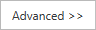button, and defining custom field stress as required. See the Custom Field Stress topic for details.